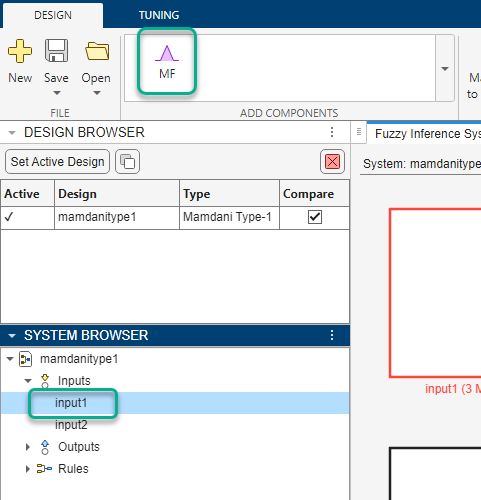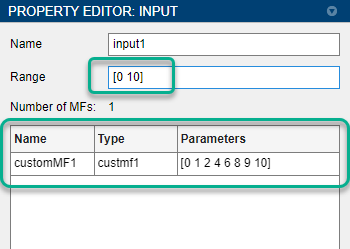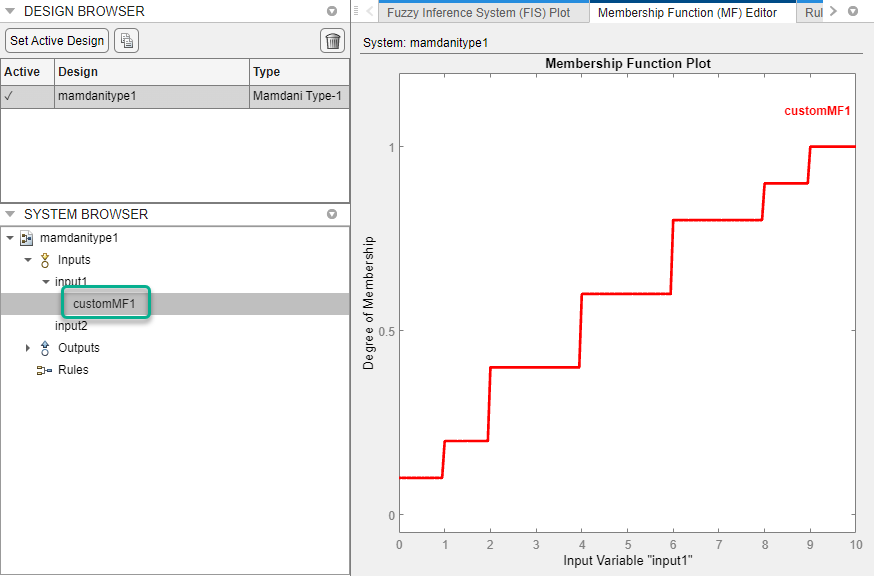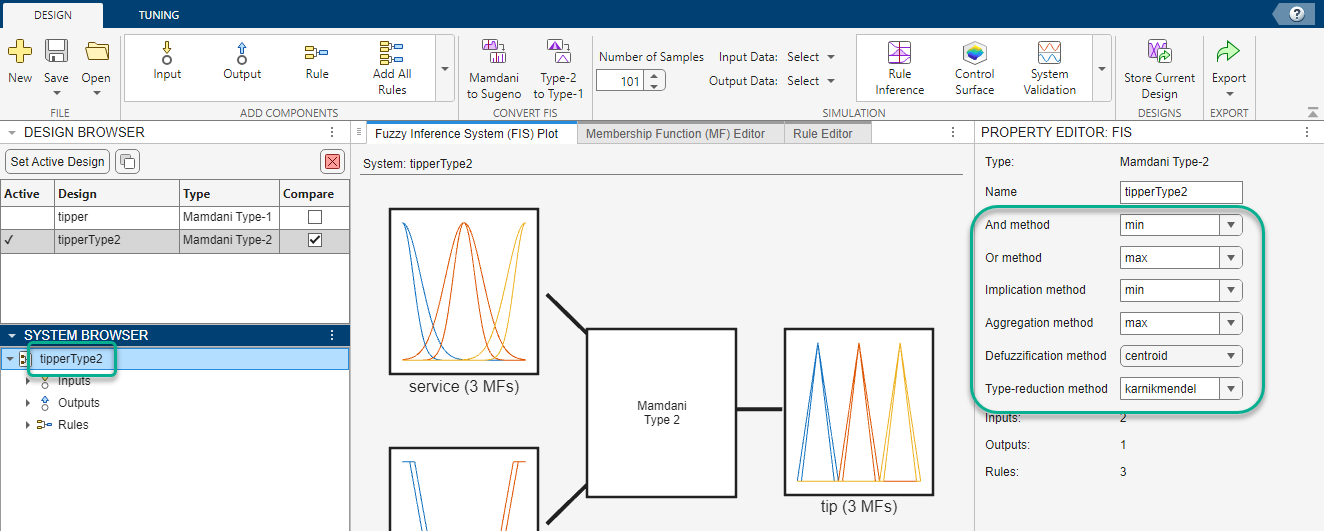## Build Fuzzy Systems Using Custom Functions

When you build a fuzzy inference system (FIS), you can replace the built-in membership functions (MFs) or inference functions with custom functions. You can create a FIS that uses these custom functions in the Fuzzy Logic Designer app and at the MATLAB® command line.

For more information on creating a FIS, see Build Fuzzy Systems Using Fuzzy Logic Designer and Build Fuzzy Systems at the Command Line.

### Define Custom Membership Functions

You can create custom membership functions and use them in the fuzzy inference process. The values of these functions must lie between 0 and 1. For more information on the properties of membership functions, see Membership Functions.

When you create a custom membership function, you must save it in your current working folder or on the MATLAB path. You can then design a FIS that uses the custom membership function at the command line or in the Fuzzy Logic Designer app.

The following is an example of a multistep custom membership function `custmf1`, which depends on eight parameters between `0` and `10`.

```% Function to generate a multi-step custom membership function % using 8 parameters for the input argument x function out = custmf1(x,params) for i = 1:length(x) if x(i) < params(1) y(i) = params(1); elseif x(i) < params(2) y(i) = params(2); elseif x(i) < params(3) y(i) = params(3); elseif x(i) < params(4) y(i) = params(4); elseif x(i) < params(5) y(i) = params(5); elseif x(i) < params(6) y(i) = params(6); elseif x(i) < params(7) y(i) = params(7); elseif x(i) <= params(8) y(i) = params(8); else y(i) = 0; end end % Scale the output to the range [0,1]. out = 0.1*y'; end```

#### Specify Custom Membership Functions Using Fuzzy Logic Designer

Since R2022b

To use a custom membership function when designing a FIS using the Fuzzy Logic Designer app, first select the corresponding variable in the System Browser. Then, in the Add Components gallery, click MF.The app adds a default triangular membership function to the selected variable.

In the Property Editor:

1. In the Range field, specify an input range that matches the expected range of your membership function.

2. In the Name column, specify a name for the membership function.

3. In the Type column, specify the name of the custom membership function.

4. In the Parameters column, enter the membership function parameters.To verify the appearance of your membership function, select the membership function in the System Browser and open the Membership Function Editor.The following features are not supported for custom membership functions:

• Interactively adjusting the parameters of a custom membership function in the Membership Function Editor.

• Automatically distributing custom membership functions across a variable range.

### Define Custom Inference Functions

Depending on the type of FIS you design, you can replace the built-in AND, OR, implication, aggregation, and defuzzification inference methods with custom functions. For each type of inference function, the following table lists the FIS objects that support using custom functions.

Inference FunctionSupported FIS Objects
ANDAll FIS objects
OR
Implication
• Type-1 Mamdani FIS

• Type-2 Mamdani FIS

Aggregation
DefuzzificationType-1 Mamdani FIS
Type-reduction
• Type-2 Mamdani FIS

• Type-2 Sugeno FIS

When you create a custom inference function, you must save it in your current working folder or on the MATLAB path. You can then design a FIS that uses the custom inference function at the command line or in the Fuzzy Logic Designer app.

#### Create Custom AND and OR Functions

The custom AND and OR inference functions must operate column-wise on a matrix in the same way as the MATLAB functions `max`, `min`, and `prod`. For example:

• For a row or column vector `x`, `min` returns the minimum element.

```x = [1 2 3 4]; min(x) ```
```ans = 1```
• For a matrix `x`, `min` returns a row vector containing the minimum element from each column.

```x = [1 2 3 4;5 6 7 8;9 10 11 12]; min(x) ```
```ans = 1 2 3 4```
For N-D matrices, `min` operates along the first non-singleton dimension.

• For two arrays, `x` and `y`, `min` returns an array that is same size as the larger of `x` or `y` with the minimum elements from `x` or `y`. Either of the input arguments can be a scalar.

```x = [1 2; 3 4]; y = [2 2; 2 2]; min(x,y) ```
```ans = 1 2 2 2```

In Fuzzy Logic Toolbox™ software:

• AND inference functions perform an element-by-element matrix operation similar to the command `min(x,y)`.

• OR inference functions perform an element-by-element matrix operation similar to the command `max(x,y)`.

#### Create Custom Implication Functions

Custom implication functions must operate in the same way as the MATLAB functions `max`, `min`, and `prod`. Your custom implication function must be a T-norm fuzzy intersection operation. For more information, see Additional Fuzzy Operators.

An implication function must support either one or two inputs because the software calls the function in two ways.

• To calculate the output fuzzy set values using the firing strength of all the rules and the corresponding output membership functions. In this case, the software calls the implication function using two inputs as follows.

`impvals = customimp(w,outputmf)`
• `w` — Firing strength of multiple rules, specified as an Nr-by-Ns matrix. Here, Nr is the number of rules and Ns is the number of samples of the output membership functions.

`w(:,j) = w(:,1)` for all j. `w(i,1)` is the firing strength of the ith rule.

• `outputmf` — Output membership function values, specified as an Nr-by-Ns matrix. `outputmf(i,:)` contains the data of the ith output membership function.

• To calculate the output fuzzy value using the firing strength of a single rule and the corresponding output membership function, for a given sample. In this case, the software calls the implication function using one input, similar to the following example.

`impval = customimp([w outputmf])`

`w` and `outputmf` are scalar values representing the firing strength of a rule and the corresponding output membership function value, for a given sample.

The following is an example of a bounded product custom implication function with binary mapping $T\left(a,b\right)=\mathrm{max}\left\{0,a+b-1\right\}$ .

```function y = customimp(x1,x2) if nargin == 1 % x1 assumed to be nonempty column vector or matrix. minVal = zeros(1,size(x1,2)); y = ones(1,size(x1,2)); for i = 1:size(x1,1) y = max(minVal,sum([y;x1(i,:)])-1); end else % x1 and x2 assumed to be nonempty matrices. minVal = zeros(1,size(x1,2)); y = zeros(size(x1)); for i = 1:size(x1,1) y(i,:) = max(minVal,sum([x1(i,:);x2(i,:)])-1); end end end```

Note

Custom implication functions are not supported for Sugeno systems.

#### Create Custom Aggregation Functions

Custom aggregation functions must operate in the same way as the MATLAB functions `max`, `min`, and `prod` and must be of the form `y = customagg(x)`. Your custom implication function must be a T-conorm (S-norm) fuzzy intersection operation. For more information, see Additional Fuzzy Operators.

`x` is an Nv-by-Nr matrix, which is the list of truncated output functions returned by the implication method for each rule. Nv is the number of output variables and Nr is the number of rules. The output of the aggregation method is one fuzzy set for each output variable.

The following is an example of a bounded sum custom aggregation function with binary mapping $S\left(a,b\right)=\mathrm{min}\left\{a+b,1\right\}$ .

```function y = customagg(x) maxVal = ones(1,size(x,2)); y = zeros(1,size(x,2)); for i = 1:size(x,1) y = min(maxVal,sum([y;x(i,:)])); end end```

Note

Custom aggregation functions are not supported for Sugeno systems.

#### Create Custom Defuzzification Functions

Custom defuzzification functions must be of the form ```y = customdefuzz(x,ymf)```, where `x` is the vector of values in the membership function input range and `ymf` contains the values of the membership function for each `x` value.

The following is an example of a custom defuzzification function.

```function defuzzfun = customdefuzz(x,ymf) total_area = sum(ymf); defuzzfun = sum(ymf.*x)/total_area; end```

Note

Custom defuzzification functions are not supported for Sugeno systems.

#### Create Custom Type-Reduction Function

For type-2 fuzzy inference systems, you can specify a custom type-reduction function. This function must be of the form `y = customtr(x,umf,lmf)`, where `x` is the vector of values in the membership function input range. `umf` and `lmf` are the respective values of the upper and lower membership function for each `x` value. The output `y` is a two-element row vector of centroids [cL,cR].

By default, type-2 Sugeno systems support only a weighted average form of type reduction. The following custom type-reduction function implements a weighted sum form of type reduction for a Sugeno system.

```function y = customtr(x,umf,lmf) y = zeros(1,2); y(1) = sum(x.*umf); y(2) = sum(x.*lmf); end```

#### Specify Custom Inference Functions Using Fuzzy Logic Designer

Since R2022b

To use custom inference functions when designing a FIS using the Fuzzy Logic Designer app, first select the FIS in the System Browser. Then, in the Property Editor, enter the name of the custom function in the corresponding inference function field.This table shows the Property Editor field for each type of inference function.

Inference FunctionProperty Editor Field
ANDAnd method
OROr method
ImplicationImplication method
AggregationAggregation method
DefuzzificationDefuzzification method
Type-reductionType-reduction method

#### Specify Custom Inference Functions at Command Line

To use custom inference functions when designing a FIS at the MATLAB command line, set the corresponding FIS object property to the custom inference function name. For example, the following command sets the aggregation function of FIS `myFIS` to the `customagg` function.

`myFIS.AggregationMethod = "customagg";`

This table shows the FIS object property for each type of inference function.

Inference FunctionFIS Object Property
AND`AndMethod`
OR`OrMethod`
Implication`ImplicationMethod`
Aggregation`AggregationMethod`
Defuzzification`DefuzzificationMethod`
Type-reduction`TypeReductionMethod`

### Use Custom Functions in Code Generation

You can use custom functions in fuzzy inference systems for which you generate code. For more information on code generation for fuzzy systems, see Deploy Fuzzy Inference Systems.

If you use a nondouble data type for your generated code, you must propagate the data type from the input arguments of your custom function to the output argument. For example, the following custom aggregation function maintains the data type of `x` in `y` using the `ones` and `zeros` functions with the `'like'` argument.

```function y = customagg(x) %#codegen maxVal = ones(1,size(x,2),'like',x); y = zeros(1,size(x,2),'like',x); for i = 1:size(x,1) y = min(maxVal,sum([y;x(i,:)])); end end```

For more information on writing functions that support C/C++ code generation, see MATLAB Programming for Code Generation (MATLAB Coder).

 Mizumoto, Masaharu. "Pictorial Representations of Fuzzy Connectives, Part II: Cases of Compensatory Operators and Self-Dual Operators." Fuzzy Sets and Systems 32, no. 1 (August 1989): 45–79. https://doi.org/10.1016/0165-0114(89)90087-0.